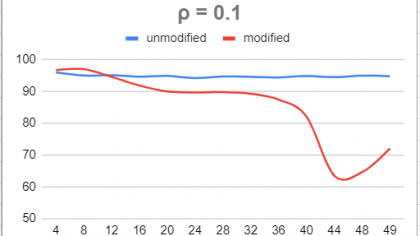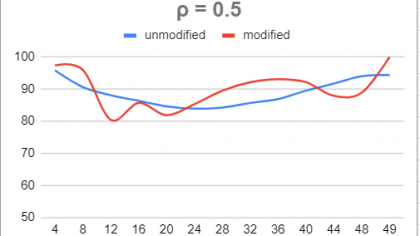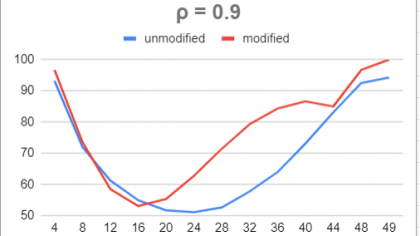## Estimation and Inference for Correlation Under Censoring

Yilun Cao '22Department of Mathematics, Ohio Wesleyan University
Faculty mentor: Scott Linder, Ph.D.

### Abstract

In many clinical and industrial settings, data are subjected to censoring. Widely used, conventional statistical methods (t-tests, linear regression, etc.) are based on underpinning sampling distributions which typically become mathematically intractable when censoring occurs. Our study examines the effect of censoring on the accuracy of the method for estimating correlation using the Fisher transformation. When censoring is imposed, we observe that actual coverage rates of confidence intervals for the population correlation coefficient constructed through the Fisher Transformation method degrade very rapidly, rendering this method inappropriate. Again using simulation, we propose a shift and scale modification to the approximate normality of the sampling distribution of the Fisher transformed sample correlation. The scale and location shifts are functions of the degree of censoring. This allows us to proposed “modified” confidence intervals for the population correlation under censoring. We observe that these modified intervals offer slight improvement in coverage rate over the unmodified version.

### Background

The Fisher Transformation is used to estimate the population correlation coefficient ρ. With r representing the sample correlation, denote the Fisher transformed sample correlation z: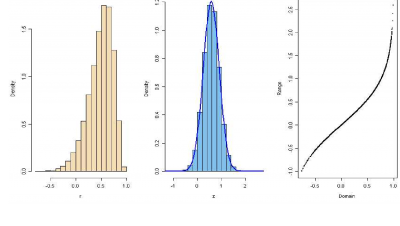z=0.5ln((1+r)/(1-r))

In the full-sample setting (no censoring), the sampling distribution of the z is approximately normal, and this approximation is quite good even for samples as small as n = 8. When p < n observations are made, censoring has occurred. Using simulation we see that this impacts the sampling distribution of z.

## METHOD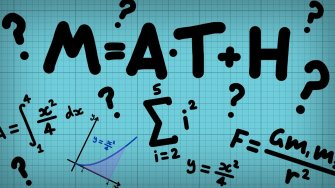## OUTCOMES

We tested the result by simulating actual confidence levels for nominally 95% confidence intervals, using n=10, 30, and 50 with ρ =0.1, 0.5, 0.9. The plots below demonstrate coverage rates when n is 50.